## Two Particles in 3 Dimensions

So far we have been working with states of just one particle in one dimension. The extension to two different particles and to three dimensions is straightforward. The coordinates and momenta of different particles and of the additional dimensions commute with each other as we might expect from classical physics. The only things that don't commute are a coordinate with its momentum, for example,whileWe may write states for two particles which are uncorrelated, like, or we may write states in which the particles are correlated. The Hamiltonian for two particles in 3 dimensions simply becomes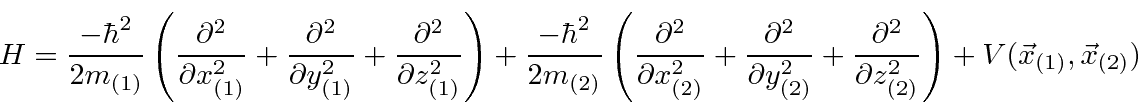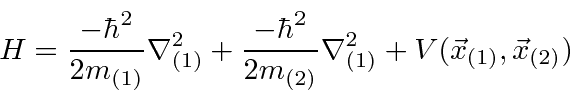If two particles interact with each other, with no external potential,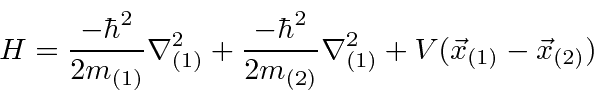the Hamiltonian has a translational symmetry, and remains invariant under the translation. We can show that this translational symmetry implies conservation of total momentum. Similarly, we will show that rotational symmetry implies conservation of angular momentum, and that time symmetry implies conservation of energy.

For two particles interacting through a potential that depends only on difference on the coordinates,we can make the usual transformation to the center of mass made in classical mechanics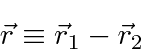and reduce the problem to the CM moving like a free particle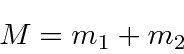plus one potential problem in 3 dimensions with the usual reduced mass.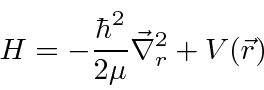So we are now left with a 3D problem to solve (3 variables instead of 6).

Jim Branson 2013-04-22